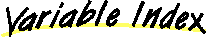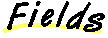`All Packages  Class Hierarchy  This Package  Previous  Next  Index`

# Class java.awt.Rectangle

```java.lang.Object
|
+----java.awt.Rectangle
```

public class Rectangle
extends Object
implements Shape, Serializable
A rectangle defined by x, y, width and height.

##height
The height of the rectangle.width
The width of the rectangle.x
The x coordinate of the rectangle.y
The y coordinate of the rectangle.

##Rectangle()
Constructs a new rectangle, initialized to location (0, 0) and size (0, 0).Rectangle(Dimension)
Constructs a rectangle and initializes it to the specified width and height.Rectangle(int, int)
Constructs a rectangle and initializes it with the specified width and height parameters.Rectangle(int, int, int, int)
Constructs and initializes a rectangle with the specified parameters.Rectangle(Point)
Constructs a rectangle and initializes it to the specified point.Rectangle(Point, Dimension)
Constructs a rectangle and initializes it to a specified point and dimension.Rectangle(Rectangle)
Constructs a new rectangle, initialized to match the values of the specificed rectangle.

##add(int, int)
Adds a point to a rectangle.add(Point)
Adds a point to a rectangle.add(Rectangle)
Adds a rectangle to a rectangle.contains(int, int)
Checks whether this rectangle contains the specified point.contains(Point)
Checks whether this rectangle contains the specified point.equals(Object)
Checks whether two rectangles are equal.getBounds()
Returns the bounds of this rectangle.getLocation()
Returns the location of this rectangle.getSize()
Returns the size (width by height) of this rectangle.grow(int, int)
Grows the rectangle horizontally and vertically.hashCode()
Returns the hashcode for this Rectangle.inside(int, int)
Deprecated.intersection(Rectangle)
Computes the intersection of two rectangles.intersects(Rectangle)
Checks if two rectangles intersect.isEmpty()
Determines whether the rectangle is empty.move(int, int)
Deprecated.reshape(int, int, int, int)
Deprecated.resize(int, int)
Deprecated.setBounds(int, int, int, int)
Set the bounds of this rectangle to the specified x, y, width, and height.setBounds(Rectangle)
Set the bounds of this rectangle to match the specified rectangle.setLocation(int, int)
Moves the rectangle to have the specificed location.setLocation(Point)
Moves the rectangle to have the specificed location.setSize(Dimension)
Set the size of this rectangle to match the specified size.setSize(int, int)
Set the size of this rectangle to the specified width and height.toString()
Returns the String representation of this Rectangle's values.translate(int, int)
Translates the rectangle.union(Rectangle)
Computes the union of two rectangles.

##x
``` public int x
```
The x coordinate of the rectangle.y
``` public int y
```
The y coordinate of the rectangle.width
``` public int width
```
The width of the rectangle.height
``` public int height
```
The height of the rectangle.

##Rectangle
``` public Rectangle()
```
Constructs a new rectangle, initialized to location (0, 0) and size (0, 0).Rectangle
``` public Rectangle(Rectangle r)
```
Constructs a new rectangle, initialized to match the values of the specificed rectangle.

Parameters:
r - a rectangle from which to copy initial valuesRectangle
``` public Rectangle(int x,
int y,
int width,
int height)
```
Constructs and initializes a rectangle with the specified parameters.

Parameters:
x - the x coordinate
y - the y coordinate
width - the width of the rectangle
height - the height of the rectangleRectangle
``` public Rectangle(int width,
int height)
```
Constructs a rectangle and initializes it with the specified width and height parameters.

Parameters:
width - the width of the rectangle
height - the height of the rectangleRectangle
``` public Rectangle(Point p,
Dimension d)
```
Constructs a rectangle and initializes it to a specified point and dimension.

Parameters:
p - the point
d - dimensionRectangle
``` public Rectangle(Point p)
```
Constructs a rectangle and initializes it to the specified point.

Parameters:
p - the value of the x and y coordinateRectangle
``` public Rectangle(Dimension d)
```
Constructs a rectangle and initializes it to the specified width and height.

Parameters:
d - the value of the width and height

##getBounds
``` public Rectangle getBounds()
```
Returns the bounds of this rectangle. This method is included for completeness, to parallel the getBounds method of Component.setBounds
``` public void setBounds(Rectangle r)
```
Set the bounds of this rectangle to match the specified rectangle. This method is included for completeness, to parallel the setBounds method of Component.

Parameters:
d - the new size for the Dimension objectsetBounds
``` public void setBounds(int x,
int y,
int width,
int height)
```
Set the bounds of this rectangle to the specified x, y, width, and height. This method is included for completeness, to parallel the setBounds method of Component.

Parameters:
width - the new width for the Dimension object
height - the new height for the Dimension objectreshape
``` public void reshape(int x,
int y,
int width,
int height)
```
Note: reshape() is deprecated. As of JDK version 1.1, replaced by setBounds(int, int, int, int).getLocation
``` public Point getLocation()
```
Returns the location of this rectangle. This method is included for completeness, to parallel the getLocation method of Component.setLocation
``` public void setLocation(Point p)
```
Moves the rectangle to have the specificed location. This method is included for completeness, to parallel the setLocation method of Component.

Parameters:
p - the new location for the pointsetLocation
``` public void setLocation(int x,
int y)
```
Moves the rectangle to have the specificed location. This method is included for completeness, to parallel the setLocation method of Component.

Parameters:
x - the x coordinate of the new location
y - the y coordinate of the new locationmove
``` public void move(int x,
int y)
```
Note: move() is deprecated. As of JDK version 1.1, replaced by setLocation(int, int).translate
``` public void translate(int x,
int y)
```
Translates the rectangle.getSize
``` public Dimension getSize()
```
Returns the size (width by height) of this rectangle. This method is included for completeness, to parallel the getSize method of Component.setSize
``` public void setSize(Dimension d)
```
Set the size of this rectangle to match the specified size. This method is included for completeness, to parallel the setSize method of Component.

Parameters:
d - the new size for the Dimension objectsetSize
``` public void setSize(int width,
int height)
```
Set the size of this rectangle to the specified width and height. This method is included for completeness, to parallel the setSize method of Component.

Parameters:
width - the new width for the Dimension object
height - the new height for the Dimension objectresize
``` public void resize(int width,
int height)
```
Note: resize() is deprecated. As of JDK version 1.1, replaced by setSize(int, int).contains
``` public boolean contains(Point p)
```
Checks whether this rectangle contains the specified point.

Parameters:
p - the point (location) to testcontains
``` public boolean contains(int x,
int y)
```
Checks whether this rectangle contains the specified point.

Parameters:
x - the x coordinate
y - the y coordinateinside
``` public boolean inside(int x,
int y)
```
Note: inside() is deprecated. As of JDK version 1.1, replaced by contains(int, int).intersects
``` public boolean intersects(Rectangle r)
```
Checks if two rectangles intersect.intersection
``` public Rectangle intersection(Rectangle r)
```
Computes the intersection of two rectangles.union
``` public Rectangle union(Rectangle r)
```
Computes the union of two rectangles.add
``` public void add(int newx,
int newy)
```
Adds a point to a rectangle. This results in the smallest rectangle that contains both the rectangle and the point.add
``` public void add(Point pt)
```
Adds a point to a rectangle. This results in the smallest rectangle that contains both the rectangle and the point.add
``` public void add(Rectangle r)
```
Adds a rectangle to a rectangle. This results in the union of the two rectangles.grow
``` public void grow(int h,
int v)
```
Grows the rectangle horizontally and vertically.isEmpty
``` public boolean isEmpty()
```
Determines whether the rectangle is empty.hashCode
``` public int hashCode()
```
Returns the hashcode for this Rectangle.

Overrides:
hashCode in class Objectequals
``` public boolean equals(Object obj)
```
Checks whether two rectangles are equal.

Overrides:
equals in class ObjecttoString
``` public String toString()
```
Returns the String representation of this Rectangle's values.

Overrides:
toString in class Object

`All Packages  Class Hierarchy  This Package  Previous  Next  Index`